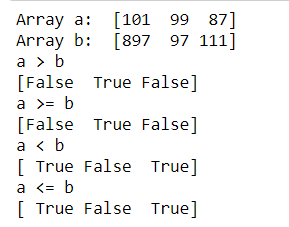Open in App
Not now

# How to compare two NumPy arrays?

• Difficulty Level : Basic
• Last Updated : 03 Jun, 2022

Here we will be focusing on the comparison done using NumPy on arrays. Comparing two NumPy arrays determines whether they are equivalent by checking if every element at each corresponding index is the same.

Method 1: We generally use the == operator to compare two NumPy arrays to generate a new array object. Call ndarray.all() with the new array object as ndarray to return True if the two NumPy arrays are equivalent.

## Python3

 `import` `numpy as np` `an_array ``=` `np.array([[``1``, ``2``], [``3``, ``4``]])``another_array ``=` `np.array([[``1``, ``2``], [``3``, ``4``]])` `comparison ``=` `an_array ``=``=` `another_array``equal_arrays ``=` `comparison.``all``()` `print``(equal_arrays)`

Output:

`True`

Method 2: We can also use greater than, less than and equal to operators to compare. To understand, have a look at the code below.

```Syntax : numpy.greater(x1, x2[, out])
Syntax : numpy.greater_equal(x1, x2[, out])
Syntax : numpy.less(x1, x2[, out])
Syntax : numpy.less_equal(x1, x2[, out])```

## Python3

 `import` `numpy as np`  `a ``=` `np.array([``101``, ``99``, ``87``])``b ``=` `np.array([``897``, ``97``, ``111``])` `print``(``"Array a: "``, a)``print``(``"Array b: "``, b)` `print``(``"a > b"``)``print``(np.greater(a, b))` `print``(``"a >= b"``)``print``(np.greater_equal(a, b))` `print``(``"a < b"``)``print``(np.less(a, b))` `print``(``"a <= b"``)``print``(np.less_equal(a, b))`

Output:## Method 3: Using array_equal()

This array_equal() function checks if two arrays have the same elements and same shape.

Syntax:

`numpy.array_equal(arr1, arr2) `

Parameters:

• arr1    : [array_like]Input array or object whose elements, we need to test.
• arr2    : [array_like]Input array or object whose elements, we need to test.

Return Type: True, two arrays have the same elements and same shape.; otherwise False

Example

## Python3

 `import` `numpy as np` `arr1 ``=` `np.array([[``1``, ``2``], [``3``, ``4``]])``arr2 ``=` `np.array([[``1``, ``2``], [``3``, ``4``]])`  `# Comparing the arrays``if` `np.array_equal(arr1, arr2):``    ``print``(``"Equal"``)``else``:``    ``print``(``"Not Equal"``)`

Output:

`Equal`

My Personal Notes arrow_drop_up